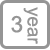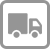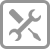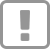• 产品详情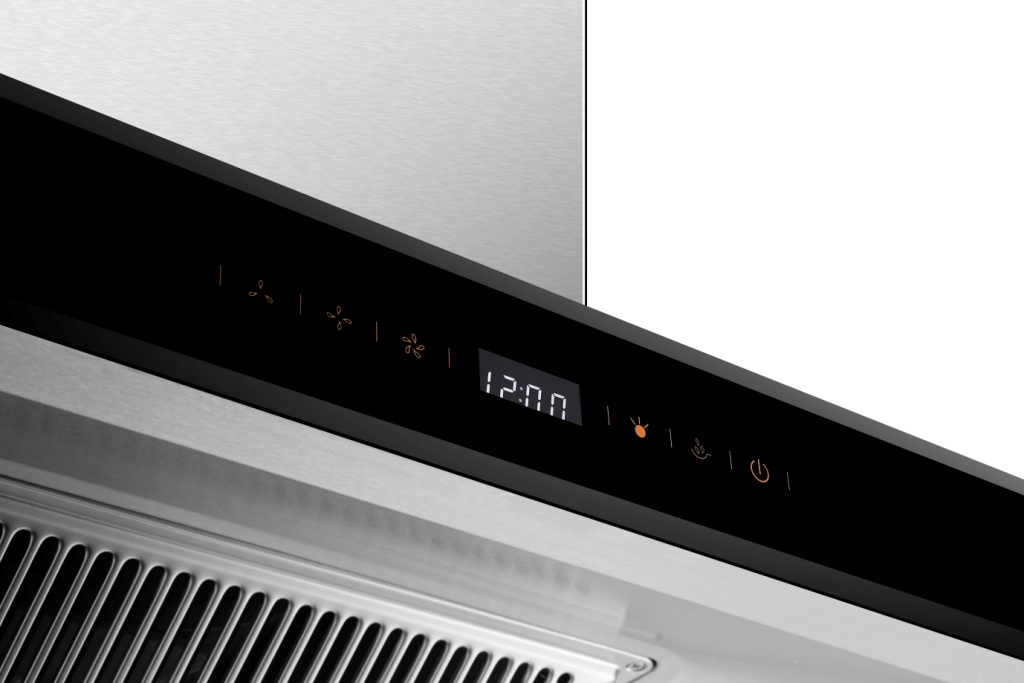^^^^^^^^^^^^^^^^^^^^^^^^^^^^^^^^^^^^^^^^^^^^^^^^^^^^^^^^^^^^^^^^^^^^^^^^^^^^^^^^^^^

5mm茶色四周磨边钢化设计，平滑时尚美观，精细化修角，拉丝纹路清晰质感，棱角线条流畅。

LED照明 光源充沛不烫手

^^^^^^^^^^^^^^^^^^^^^^^^^^^^^^^^^^^^^^^^^^^^^^^^^^^^^^^^^^^^^^^^^^^^^^^^^^^^^^^^^^^

>

Professional security care for a better life

【享受服务】